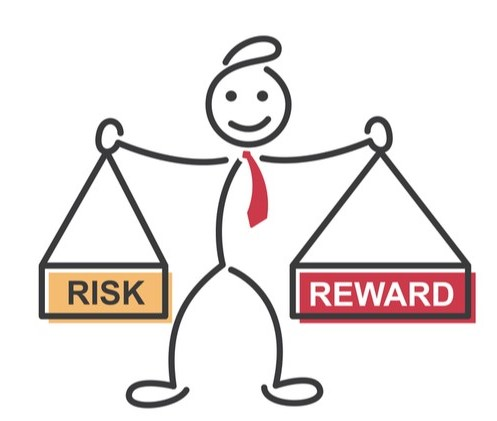## Topic outline

• ### 1. Introduction

Welcome to "Risk, Return, and the Cost of Capital!" The module analyzes the trade-off between risk and return and its implications for the practical estimation of discount rates.##### The main learning goals of this module are:
• Understand the trade-off between risk and return.
• Learn how we can reduce the risk of an asset via diversification.
• Appreciate the basics of modern portfolio theory.
• Get to know the Capital Asset Pricing Model (CAPM) to estimate expected returns.
• See how to obtain the Weighted Average Cost of Capital, the most relevant discount rate in project and firm valuation.
•View